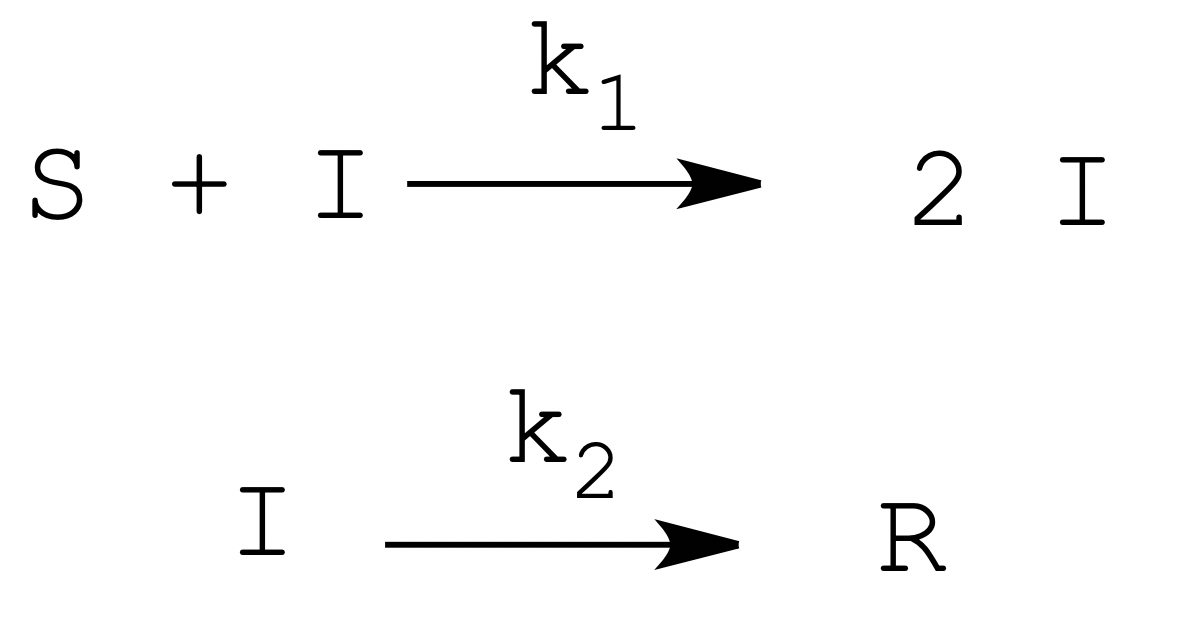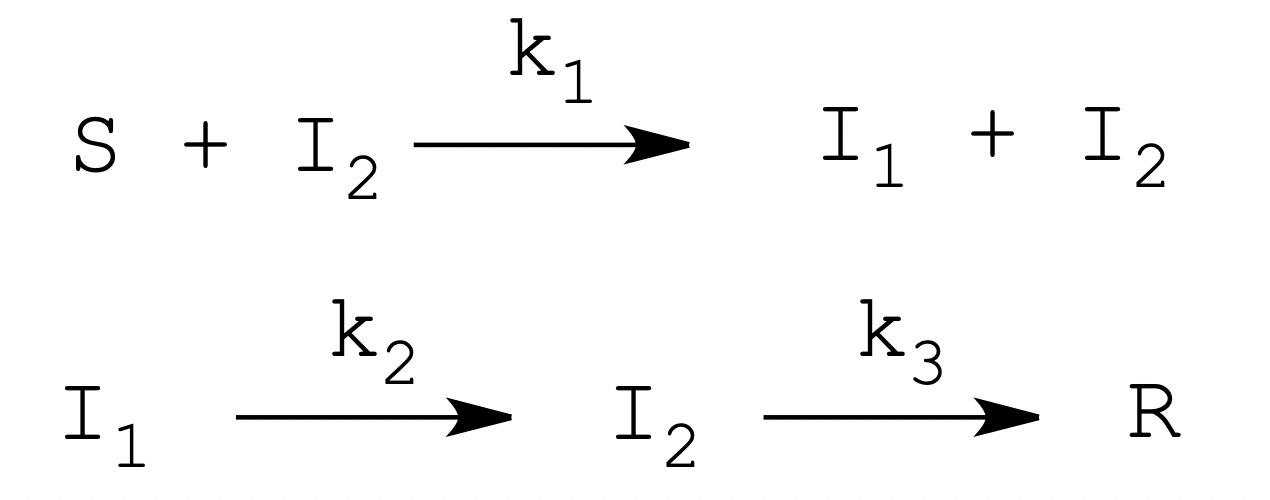Epidemics and autocatalytic biochemical processes share common features

A pedagogical exercise exploring some similarities between epidemics and biochemical processes, that may allow biochemists to obtain a semi-quantitative grasp of the basic aspects of epidemiology.

Andrea Bellelli & Francesco Malatesta
Department of Biochemical Sciences "A Rossi Fanelli"
Sapienza University of Rome

Epidemiology is quite distant from biochemistry and requires a highly specialized approach. Nevertheless, there are some similarities between epidemics and some biochemical processes, that may allow biochemists to obtain a semi-quantitative grasp of the basic aspects of epidemiology and may be worth investigating at least for pedagogical purposes.

An epidemic resembles an autocatalytic biochemical process similar to the self-activation of proteolytic enzymes. In these processes the substrate is a precursor of the enzyme and is catalytically converted to the enzyme itself, in a reaction that is reminiscent of contagion. A similar biochemical event is the stabilization of the insoluble state of the prion protein by unfolded prion protein.

If we focus our attention to a proteolytic activation such as that of trypsinogen by trypsin, we realize that in order to better mimic an epidemic process, we should postulate a mechanism of self-inactivation of the trypsin analogous to healing or death of an infected patient. Let's hypothesize a system in which the stable enzyme percurSor (S) is catalytically activated by the actIve enzyme (I), which however is unstable and quickly becomes denatuRed (R). This reaction scheme may be modeled as a two-step mechanism:The biochemically oriented reader may be perplexed by our choice of chemical symbols: indeed, we used the standard epidemiology abbreviations for Susceptible, Infected and Recovered (or Removed) (1, 2).

The above model is governed by the second order rate constant k1 and the first order rate constant k2. Both reactions might be described by better approximations (and we shall suggest one later on) but for the pedagogical purpose of applying one's competence to the understanding of a problem for a nearby scientific field we feel that the above approximation is sufficient.

What happens when one applies the above two-step model to a population of enzyme precursors or of susceptible individuals? Initially one observes an exponential growth of the population of I; at some point, however, the rate of increase will decrease because of the consumption of S, and the system will enter a condition similar to a steady state, in which the rate of formation of I equals that of its decay to R. The steady state ends when the rate of the conversion of I to R exceeds that of formation of I. This time course is analogous to that of the epidemic, in which the infected people increase, peak and decrease again because of the recovery rate exceeding that of infection. Notice that the analogy is based on the statistical mechanisms governing the two processes of proteolytic cascade and epidemic and occurs at deeper level than the mere similarity of two functions.

Since the denaturation of the activated enzyme is first order, at some point it is bound to exceed the rate of zymogen activation, which is second order. As a consequence, depending on the values of the kinetic rate constants, it may happen that the system reaches and end point in which no molecule of I is present, but some S has remained: this is also the mechanism by which an epidemic ends without affecting all the susceptible individuals in the population. The attack rate of the epidemic is defined as the fraction of individuals converted from state S to state R, and approximately corresponds to the fraction of immune individuals required to protect the susceptible ones from contagion (the herd immunity). We do not use these concepts in enzymology, and since the denaturation of the activated enzyme is usually low, the attack rates of proteolytic cascades in a test tube usually approach unity; however, we feel confident that these concepts may be easily grasped by biochemistry students.

Can we improve the above model, and do we want to? In principle, given its purely pedagogical scope, there would be no need to improve the model. However, there is a very simple addition that may make it more compelling and we feel that introducing it is worthwhile. Let's thus suppose that the activation of the enzyme precursor is a two-step process, in which the first step is proteolytic cleavage and the second step is a first order conformational rearrangement this requires two different states of I that we call I1 and I2. Our model becomes:This model requires three kinetic rate constants, of which k1 is second order, whereas k2 and k3 are first order. In this model I1 is inactive, and only I2 has full catalytic competence. The addition of state I1 has two interesting effects: (i) the course of the "molecular disease" from I1 to R is non-exponential and may more closely resemble that of human diseases; and (ii) the conversion of I1 to I2 mimics the incubation period of the disease and introduces a delay between "contagion" and "infectivity", the non-zero serial generation time of the molecular epidemic. Obviously, from the epidemiological point of view state R is the state in which infectivity - catalytic activity is lost, rather than the actual end point of the molecular disease.

We view this line of reasoning as a pedagogical exercise, and invite the interested teacher-student to visit a web-based resource that simulates the above scheme at the following link:

References

1) W.O. Kermack, A.G. McKendrick
A contribution to the mathematical theory of epidemics
Proc. R. Soc. London, 115 (1927) 700

2) F. Brauer
The Kermack–McKendrick epidemic model revisited
Mathematical Biosciences 198 (2005) 119–131

Francesco Malatesta

Professor of Chemistry and Biochemistry, francesco.malatesta@uniroma1.it